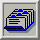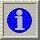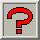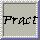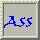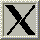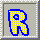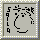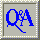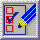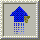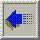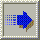##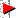3.16. Dithering

From Heckbert (1982)

The basic strategy of dithering is to trade intensity resolution for spatial resolution. That is, by averaging the intensities of several neighbouring pixels, one can get colours not represented by the colourmap. If the resolution we are using is high enough, the eye will do the spatial blending for us. Taking advantage of this, it is possible to reproduce many colour images using only four colours.

The dithering technique looked at here is due to Floyd and Steinburg (1975). Their algorithm compensates for the quantisation error introduced at each pixel by propagating it to its nearest neighbours. If the propagation is directed only to pixels below or to the right of the "current pixel", we can do both quantisation and propagation in one top-to-bottom pass over the image. The code of such an algorithm would look something like this: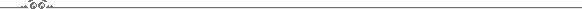```for i=0 to NI-1 do
for j=0 to NJ-1 do
begin
x = c(i,j);                       (read a colour)
k = p(x);                         (find nearest rep.)
f(i,j) = k;                       (draw quantised image)
e = x - y(k);                     (quantisation error)

(distrib. in 3 directions)
c(i,j+1) = c(i,j+1) + e*3/8;
c(i+1,j) = c(i+1,j) + e*3/8;
c(i+1,j+1) = c(i+1,j+1) + e/4;
end
```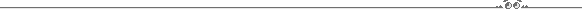Further reading on various dithering techniques can be found in Jarvis et al. (1976).